# Modulus And Argument Of Complex Numbers

We will first define two useful quantities related to an arbitrary complex number $$z = x + iy.$$

($$a) \;\;\fbox{\({{\rm{Modulus:}}\,\,\,\,\,\left| z \right|}$$}\)

The modulus, which can be interchangeably represented by $$\left| z \right|$$ or $$r,$$ is the distance of the point $$z$$ from the origin, so that its numerical value is given by $$\left| z \right| = r = \sqrt {{x^2} + {y^2}}$$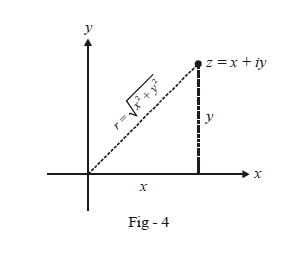(b)  $$\fbox{\({{\rm{Argument:}}\,\,\,\,\,\arg \left( z \right)}$$}\)

The argument of $$z$$ represented interchangeably by arg$$(z)$$ or $$\theta$$  , is the angle that the line joining $$z$$ to the origin makes with the positive direction of the real axis. The argument of $$z$$ can have infinite possible values; this is because if $$\theta$$  is an argument of $$z,$$ then $$2n\pi + \theta$$  is also a valid argument.

To be more specific, we define a unique value called the principal argument of $$z.$$ The convention that is used to determine the principal argument for the four different quadrants in which $$z$$ can lie, is depicted below: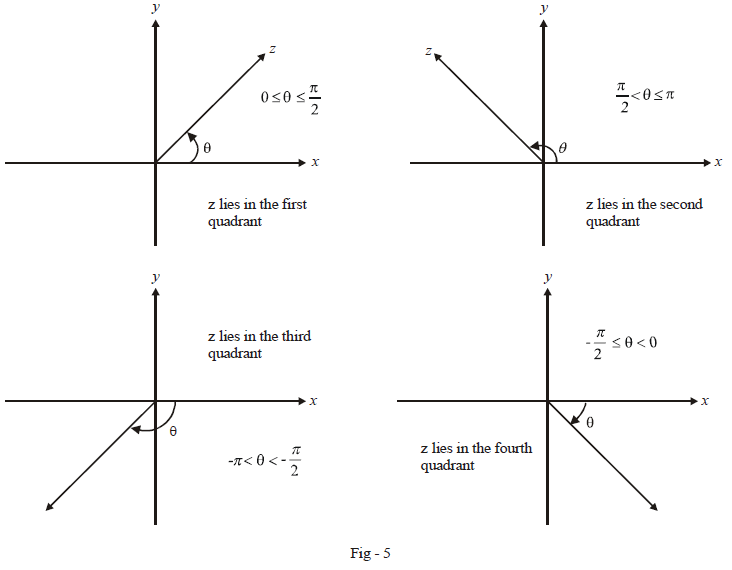Notice that Principal arg$$\left( z \right) \in \left( { - \pi ,\,\,\pi } \right]$$ . For $$z$$ above or on the real axis, principal arg $$(z)$$$$\in \,\,[0,\,\pi ]$$; it is non-negative and measured in the anti-clockwise direction from the positive real axis. For $$z$$ below the real axis, principal arg$$\left( z \right) \in \left( { - \pi ,\,\,0} \right);$$ it is negative and measured in a clockwise direction from the positive real axis.

From now on, arg$$(z)$$ will be considered to mean Principal arg$$(z)$$.

Observe now that we have two ways to specify an arbitrary complex number; one is the standard way $$(x, y)$$ which is referred to as the Cartesian form of the point. The second is by specifying the modulus and argument of $$z,$$ instead of its $$x$$ and $$y$$ components  i.e., in the form $$\left( {r,\,\,\theta } \right)$$ . This is referred to as the Polar form of the point.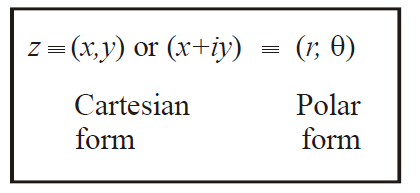Example - 1

Plot the following points on a plane and evaluate their polar forms:

 (a) $$z = 1 + i$$ (b)  $$z = - 1 + 2i$$ (c) $$z = - 3 - 3i$$ (d) $$z = 3 - 4i$$ (e) $$z = 2i$$ (f) $$z = 3$$ (g) $$z = - 4$$ (h)  $$z = - 5i$$

Solution:  Observe the figures drawn for each of these parts carefully to determine how $$\left( {r,\,\,\theta } \right)$$ is evaluated from  $$\left( {x,y} \right)$$ or $$x + iy.$$  The polar forms so obtained are boxed.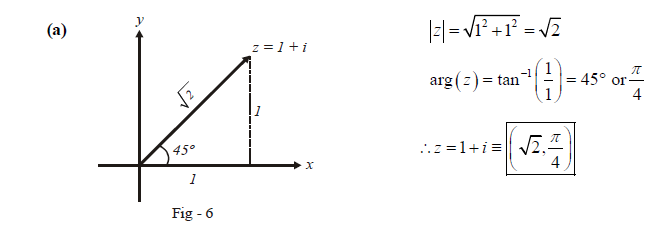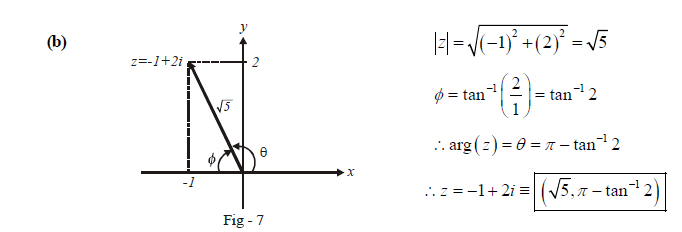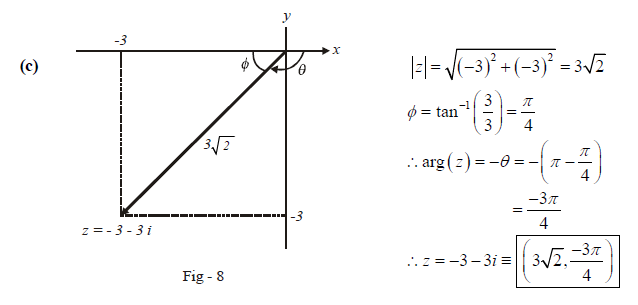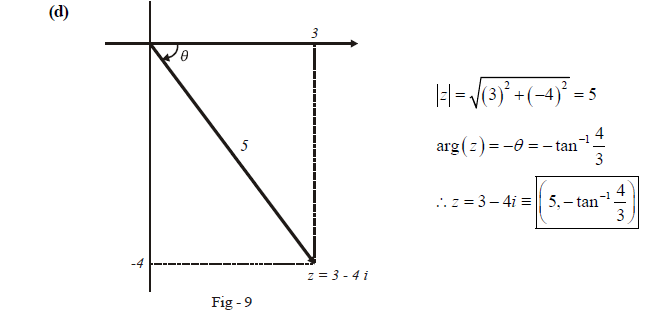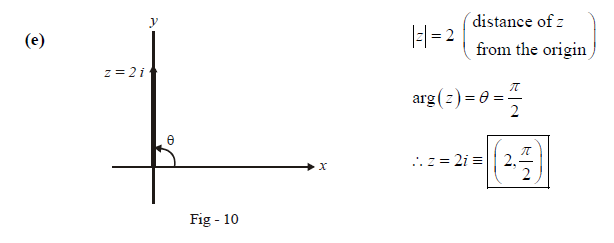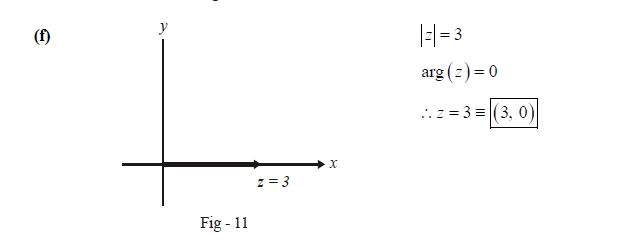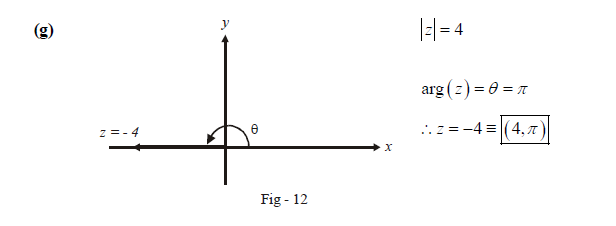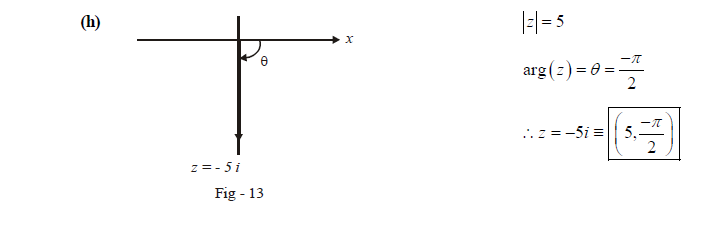Parts $$(f)$$ and $$(g)$$ above were included particularly so that you develop a tendency of thinking of even purely real numbers as points on the plane, and realise the fact that the real set $$\mathbb{R}$$  is just a subset of $$\mathbb{C}.$$

Before moving to arithmetic operations on complex numbers, observe one more important relation between the cartesian and polar form of a complex number. You have seen how to write the polar form from the cartesian form. How do we do the reverse? This is quite straight forward: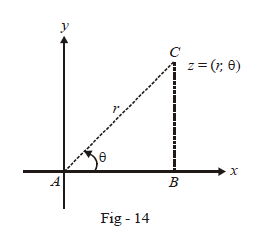Observe that $$AB = r\cos \theta \,\,\,{\rm{and}}\,\,\,BC = r\sin \theta$$.

Therefore, the cartesian form is simply$$\left( {r\cos \theta ,\,\,r\sin \theta } \right)$$ or $$r\cos \theta + \,\,i\,r\sin \theta$$ .

$z = \left( {r,\theta } \right)\,\, \equiv \,\,\left( {r\cos \theta ,\,\,r\sin \theta } \right) \equiv r\left( {\cos \theta + i\sin \theta } \right)$

The representation

$z = r\left( {\cos \theta + i\sin \theta } \right)$

is extremely useful, as we will soon see.

## TRY YOURSELF - I

Q. 1  Plot the following complex numbers on a plane and evaluate their moduli and principal arguments.

 (a)  $$z = 1 + 7i$$ (b) \begin{align}z = - \frac{1}{2} + \frac{{\sqrt 3 }}{2}\;i\end{align} . (c)  \begin{align}z = \frac{1}{{\sqrt 2 }} - \frac{1}{{\sqrt 2 }}i\end{align} (d) $$z = - 12 - 5\;i$$ (e) $$z = 6 - 7\;i$$ ( f) \begin{align}z = {\left( {\frac{{i - 1}}{{i + 1}}} \right)^3}\end{align}

Q. 2  Evaluate  $${i^{996}} + {i^{.997}} + {i^{998}} + {i^{.999}}$$

Q. 3  For what smallest positive integral value of $$n$$ is \begin{align}{\left( {\frac{{1 + i}}{{1 - i}}} \right)^n}\end{align} equal to 1 ?

Q. 4  What is the mistake in this computation?

$1 = \sqrt 1 = \sqrt {( - 1) \times ( - 1)} = \sqrt { - 1} \times \sqrt { - 1} = i \times i = - 1$

Learn from the best math teachers and top your exams

• Live one on one classroom and doubt clearing
• Practice worksheets in and after class for conceptual clarity
• Personalized curriculum to keep up with school